# Binary Lesson 4 Hexadecimal and Binary Practice Counting

• Slides: 17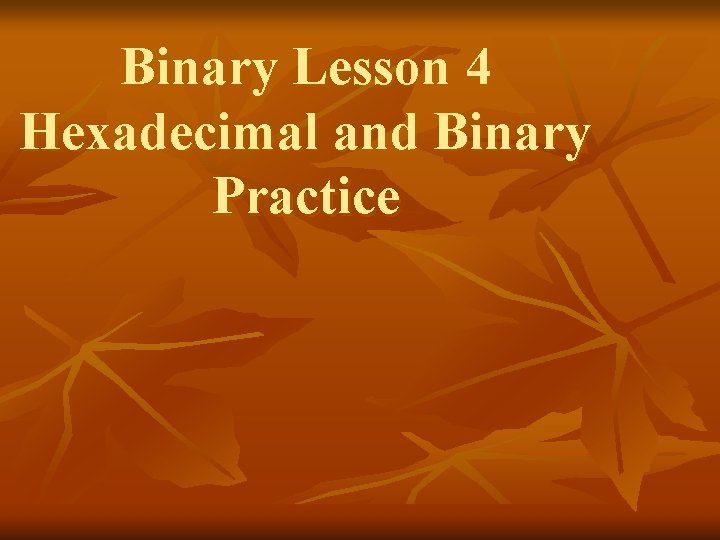Binary Lesson 4 Hexadecimal and Binary Practice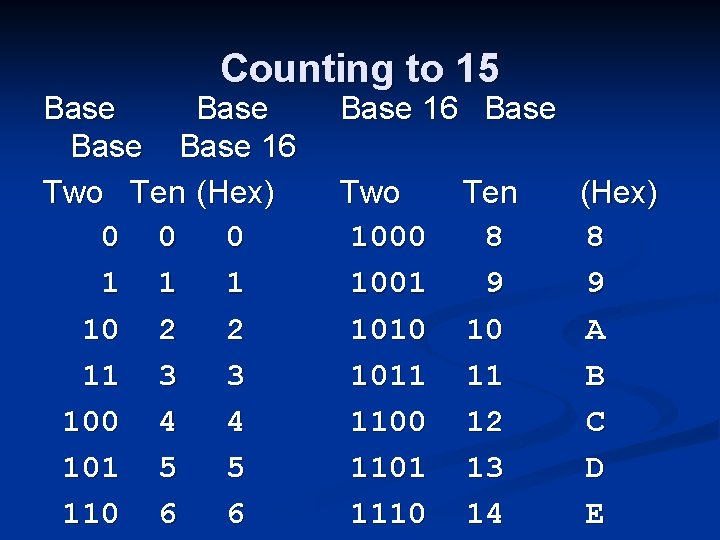Counting to 15 Base 16 Two Ten (Hex) 0 0 0 1 10 2 2 11 3 3 100 4 4 101 5 5 110 6 6 Base 16 Base Two 1000 1001 1010 1011 1100 1101 1110 Ten 8 9 10 11 12 13 14 (Hex) 8 9 A B C D E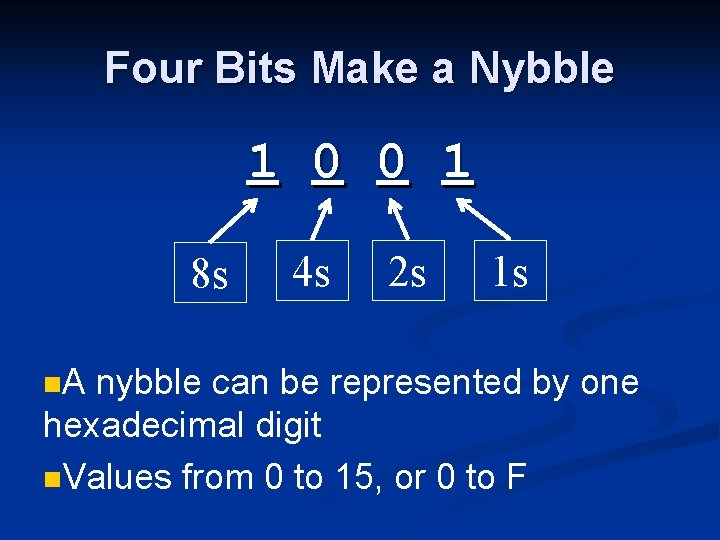Four Bits Make a Nybble 1 0 0 1 8 s n. A 4 s 2 s 1 s nybble can be represented by one hexadecimal digit n. Values from 0 to 15, or 0 to F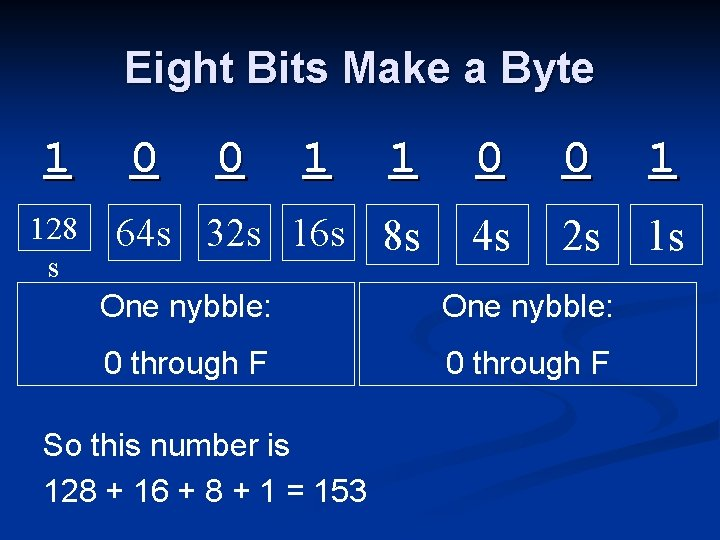Eight Bits Make a Byte 1 128 s 0 0 1 1 0 0 1 64 s 32 s 16 s 8 s 4 s 2 s 1 s One nybble: 0 through F So this number is 128 + 16 + 8 + 1 = 153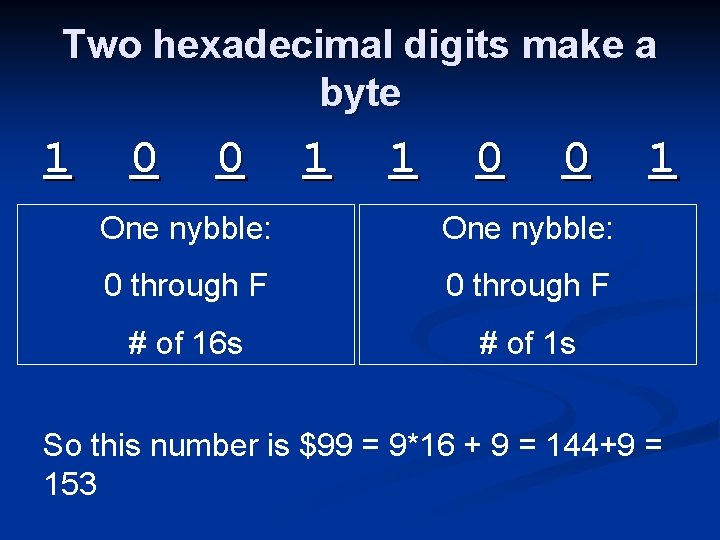Two hexadecimal digits make a byte 1 0 0 1 1 0 0 One nybble: 0 through F # of 16 s # of 1 s 1 So this number is \$99 = 9*16 + 9 = 144+9 = 153Binary i. Clicker Questions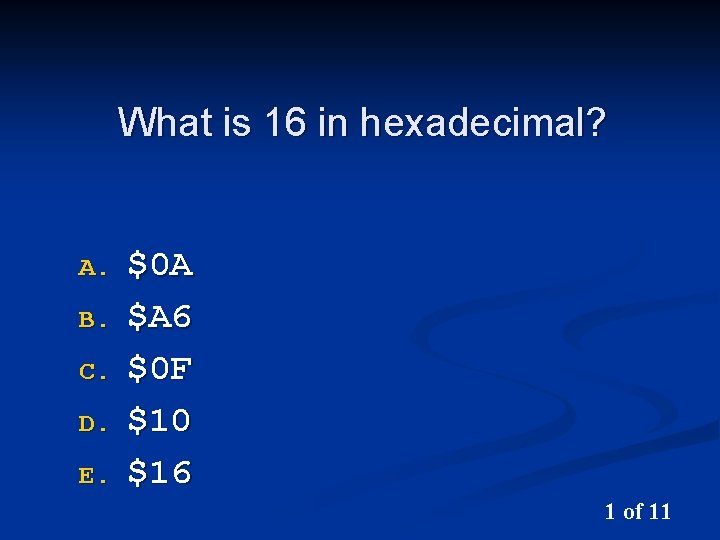What is 16 in hexadecimal? A. B. C. D. E. \$0 A \$A 6 \$0 F \$10 \$16 1 of 11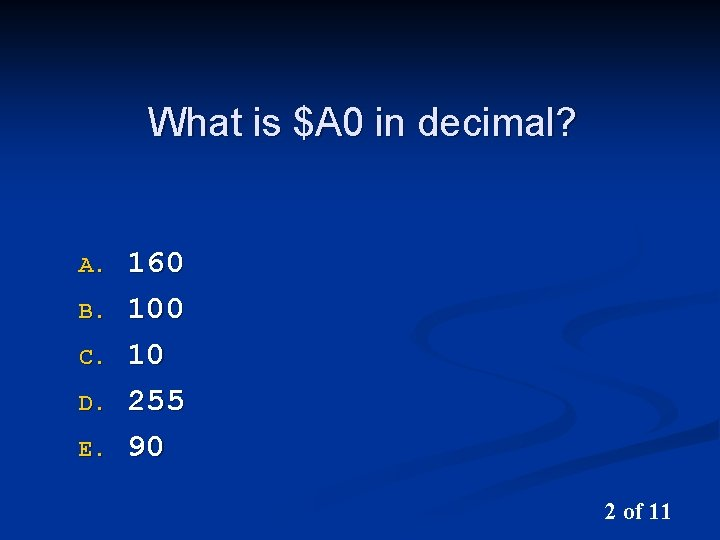What is \$A 0 in decimal? A. B. C. D. E. 160 10 255 90 2 of 11What is 64 in hexadecimal? A. B. C. D. E. \$20 \$34 \$40 \$44 3 of 11What is 252 in hexadecimal? A. B. C. D. E. \$F 0 \$F 8 \$FA \$FC \$FE 4 of 11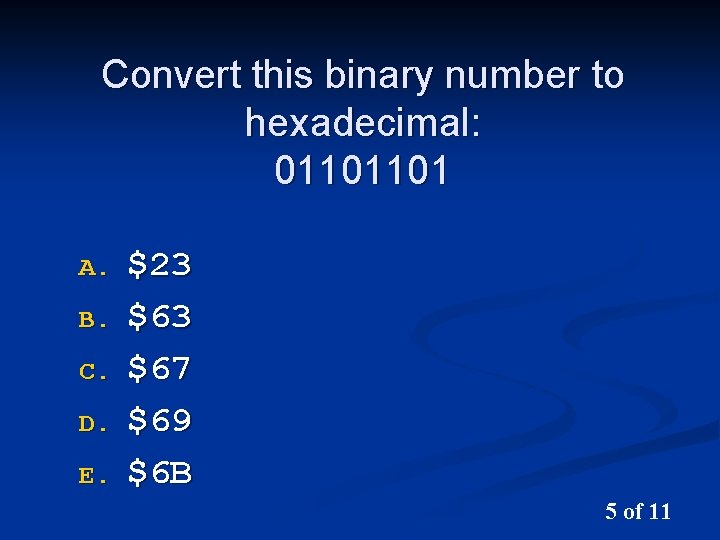Convert this binary number to hexadecimal: 01101101 A. B. C. D. E. \$23 \$67 \$69 \$6 B 5 of 11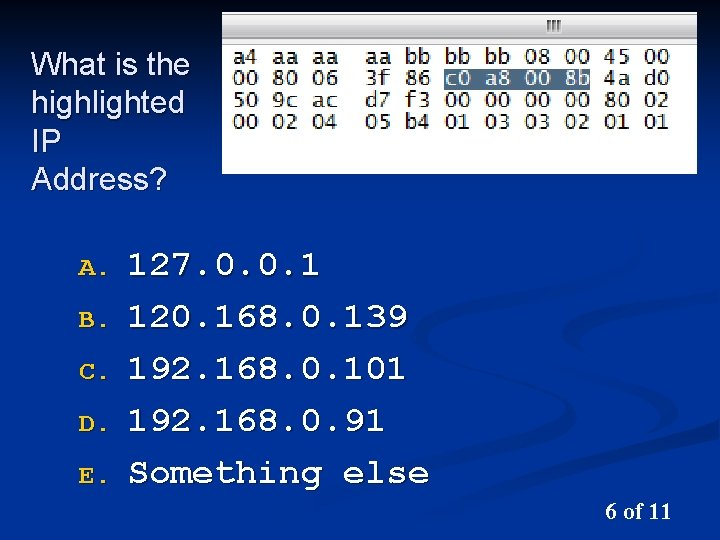What is the highlighted IP Address? A. B. C. D. E. 127. 0. 0. 1 120. 168. 0. 139 192. 168. 0. 101 192. 168. 0. 91 Something else 6 of 11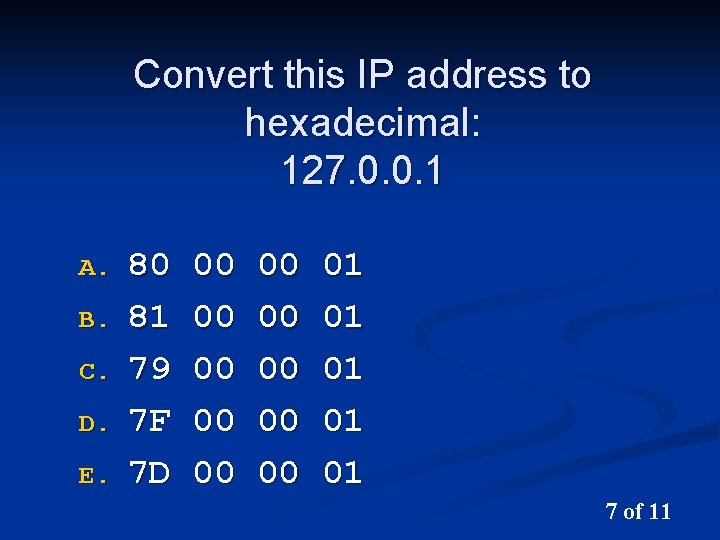Convert this IP address to hexadecimal: 127. 0. 0. 1 A. B. C. D. E. 80 81 79 7 F 7 D 00 00 00 01 01 01 7 of 11Convert this IP address to binary: 127. 0. 0. 1 A. B. C. D. E. 1111 01111111 00011111 00000000 00000000 000000001 00000001 8 of 11Convert this IP address to binary: 147. 144. 0. 1 A. B. C. D. E. 1111 01111111 10010011 10010111 00000000 10010000 10110000000000000001 00000001 9 of 11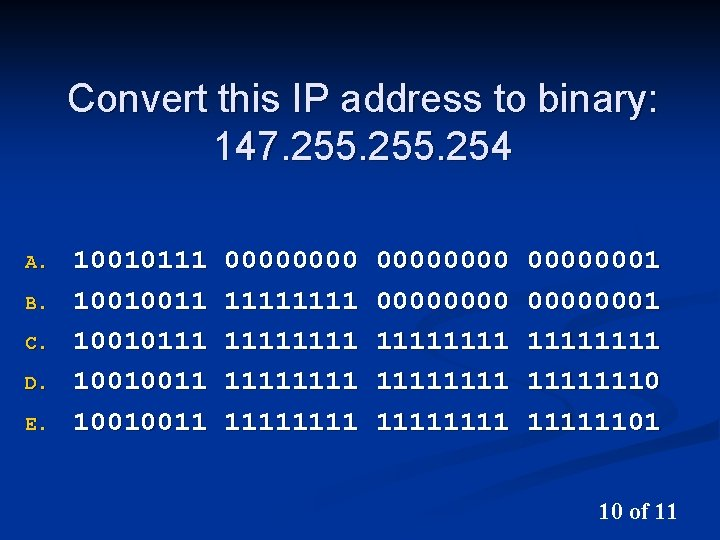Convert this IP address to binary: 147. 255. 254 A. B. C. D. E. 10010111 10010011 0000 11111111 00000000 11111111 00000001 11111110 11111101 10 of 11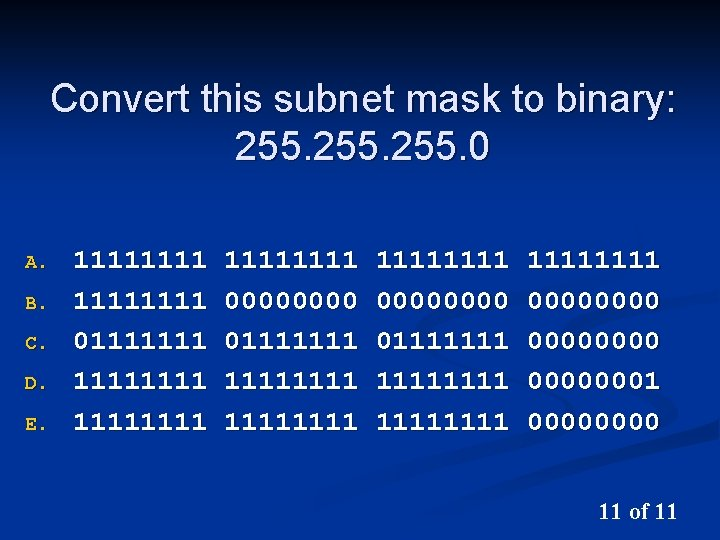Convert this subnet mask to binary: 255. 0 A. B. C. D. E. 11111111 011111111 11111111 00000000 011111111 1111 000000001 0000 11 of 11